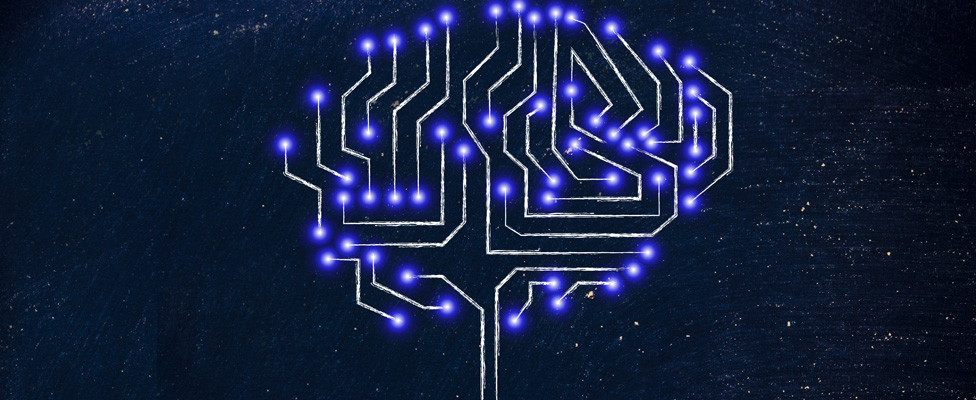### Data Digest: Today's and Tomorrow's Machine Learning Fundamentals

These articles explore the basic definitions of data science and ML, the details of ML engineering, and the potential of quantum ML.

#### Basics of Data Science and ML

The similarities and differences among machine learning, data science, and data analytics.

#### Basics of ML Engineering

Are you interested in becoming a machine learning engineer?

#### Basics of Quantum Machine Learning

Is quantum machine learning the future of ML?